# Pixel-Level Collision Detection for Transformed GraphicsThis post is part of a series called Collision Detection and Reaction.
Quick Tip: Collision Reaction Between a Circle and a Line Segment
Pixel-Level Collision Detection

In the previous tutorial, we went through the basics of pixel-level collision detection. In this tutorial, we shall explore the use of matrices in better defining the area of interest - very useful for graphics that have been rotated, translated, or skewed.

## Collision Detection

This is the final piece we will try to progam. Click on the ball and hook to start the interactive demo.

Notice how, despite the coconut tree graphic rotating and being otherwise transformed, we still have pixel-perfect collision detection.

## Step 1: The draw Function

Performing pixel-level collision detection requires the graphic to be cast to a BitmapData object. We have looked at this in the previous tutorial by using the analogy of an x-ray. In this tutorial, I shall explain this "x-ray"-ing process.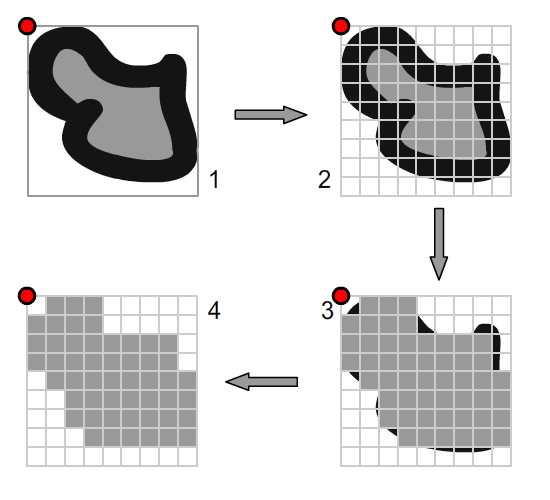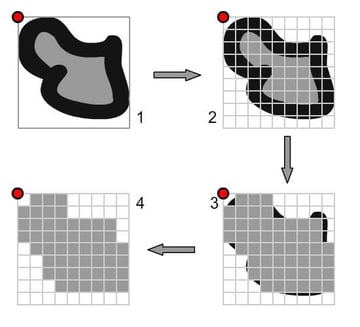Suppose we have a piece of a graphic (diagram 1) and wanted to cast it to a BitmapData object; we need to define the dimensions of the BitmapData object first (diagram 2). In this case, it's quite simple because the graphic's width and height properties provide this. Then we call the draw() method; pixels that are at least half-occupied by the graphic will be filled up, in theory (diagram 3). This array of pixels will be compared against yet another array of pixels from another graphic to check for a collision between the two (diagram 4).

## Step 2: Different Coordinate Spaces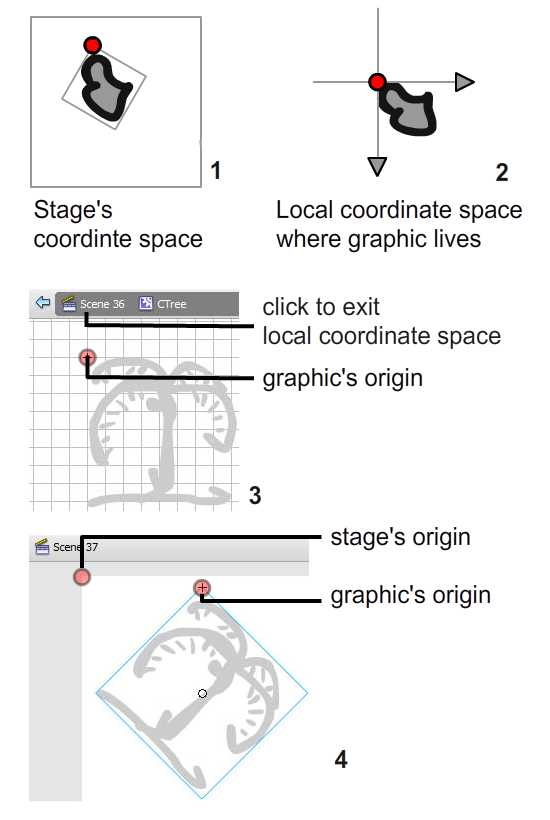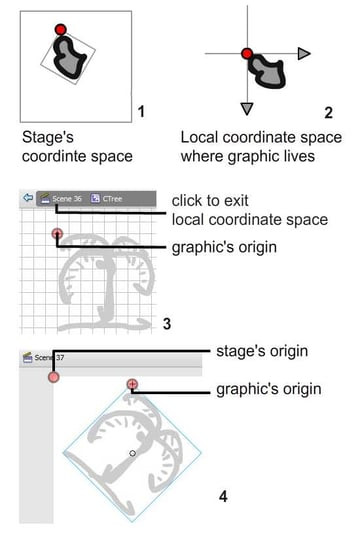There are different coordinate spaces used in Flash IDE (diagrams 1 and 2 above). I'm sure every reader would have experienced this - see my tutorial on affine spaces for a more detailed look.

In the Flash IDE, we draw an image and turn it into a symbol of type MovieClip. When we double click on the MovieClip, we arrive at a different coordinate space (diagram 3). From here, we can click on the stage label to exit this graphic's local coordinate space and arrive at the stage's coordinate space. Then we can start to transform the instance of MovieClip on the stage (diagram 4). In fact we can create multiple instances of the MovieClip, each of them having different transformations.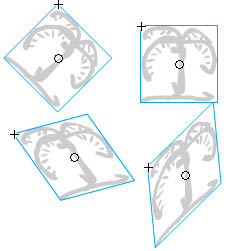In the Library, the original graphic remains unchanged despite all the tweaks done upon its copies on the stage. This is rational because whenever we make a new copy of a MovieClip on stage, they are always the same as the original copy in the library. Now the question is, "How does Flash capture all the transformation we've done to the copies on the stage?" Well, they each use the MovieClip.transform.matrix property to capture all your transformations (translation, rotation, skew, etc).

Now let's fall back to where we left off. It is crucial that we understand this because the draw() method of BitmapData does not refer to the graphic instance on the stage when performing an "x-ray", but rather to the unchanged graphic source in the library.

The BitmapData object's first pixel aligns with the registration point (the red dot) of the MovieClip on the local coordinate space (refer to diagram 3 in Step 1), then captures the graphic in pixel form with the dimensions we specify.

When it comes to hitTest checks, ActionScript aligns this first pixel (the top-left pixel) of the BitmapData object with the registration point of the graphic instance on the stage. With this, all pixels in the BitmapData object will be mapped onto the coordinate space on the stage and obtain their individual coordinates. Checks can later be performed by comparing these coordinates between two bitmaps to see if any pixels overlap.

Note: This explanation assumes the MovieClip or Sprite instance is added to the stage's display list. In ActionScript, we can actually add display objects to the document class itself because it extends MovieClip or Sprite.

## Step 3: The Problem

So if the graphic of interest is rotated on the stage, how do we perform draw()?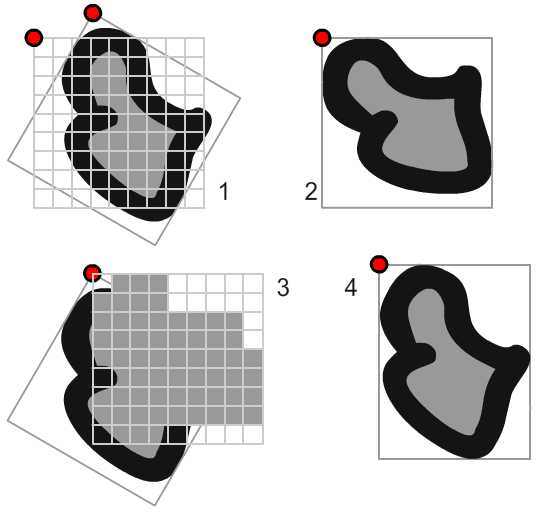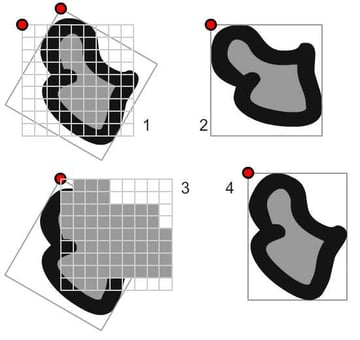From the above diagram, we can clearly see these problems.

 Diagram Problem Description 1 Dimension of BitmapData object Since the object orientation has changed, the required dimension of BitmapData cast can no longer be conveniently taken from the width and height properties of the graphic instance. 2 Orientation of graphic source The instance of the graphic on the stage is rotated, but the one in the library is not. We need to take a snapshot of the transformed graphic source from the library. 3 Coordinate of top left pixel (starting pixel) of BitmapData object We can't align the BitmapData object's first pixel with the registration point of graphic instance. This will be incorrect.

## Step 4: The Solution

To solve these problems, we will first define a rectangle tightly bounding the rotated graphic instance on the stage. ActionScript provisions for this through the getBounds() function. This will solve our first problem. Observe the image below. Notice that there's a difference between the graphic instance's registration point and that of the rectangle.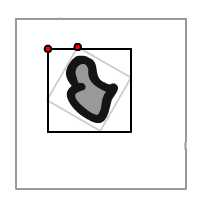I have included the Flash presentation below to show the rectangular bounding box (red Box) and the local space bounding box (black box)

## Step 5: Transformation

Next, we shall bring a snapshot of the transforrmed graphic source into this rectangle on stage. Observe the image below.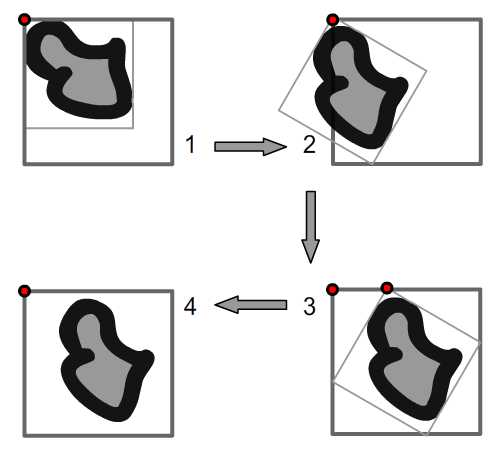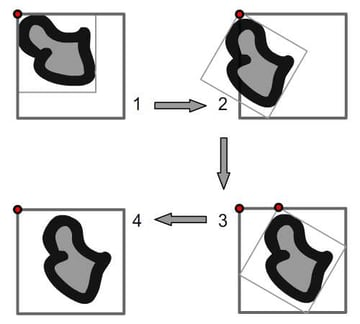We start out having the registration point of the graphic source aligned with that of the rectangular area (diagram 1). Then, we rotate (diagram 2) and offset it (diagram 3) before taking the "x-ray" capture of the image onto the BitmapData object (diagram 4).

We can do this manually, or choose to make a copy of the graphic instance's transform.matrix property. When using the second approach, we should be careful not to use the transform.matrix translation property - otherwise, the registration points will not align as you see in diagram 1. In both cases, we'll need to calculate the x and y distance to offset.

## Step 6: ActionScript Implementation

After this lengthy explanation, I hope it's easier to understand the code. I have highlighted the important lines and added comments:

Also, not to forget to change the location of the first pixel (top left) in BitmapData object to that of the rectangular box

And here's an example of the work.

## Step 7: Constant Update

If the shape of interest, in this case the coconut tree, is constantly transforming (rotating, scaling, shrinking, skewing, etc) then the BitmapData object has to be updated on each frame and this will take some processing. Also, note that I have opted for the alternative approach mentioned in Step 4. Here's the script to update the x-ray copy of the graphic for each frame:

The following function is performed every frame:

And this is the output. Start dragging the hook to see the animation.

## Conclusion

I understand this tutorial may not be too quick to read, but it's crucial to gain a clear understanding of what's happening. Hope this has been helpful and if you are interested in manipulating this 2x2 matrix more, visit my article on the subject. Thanks.